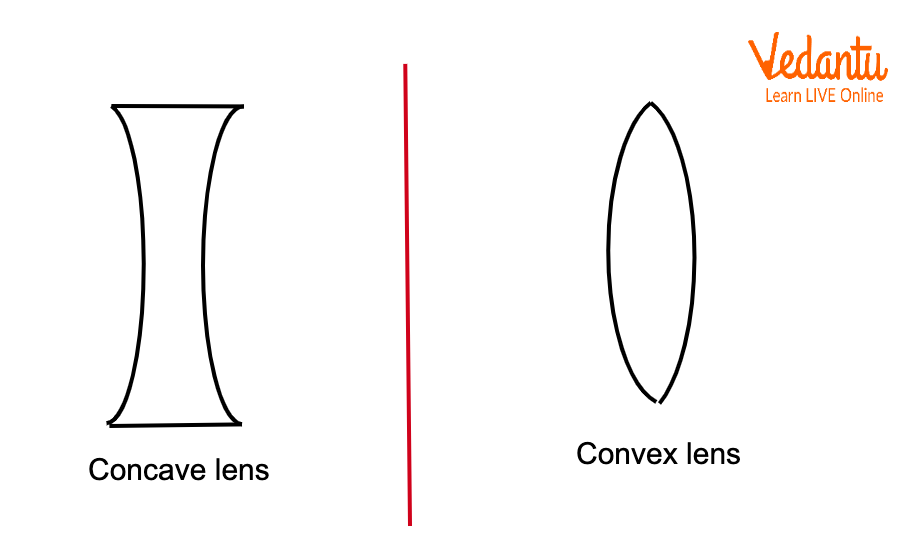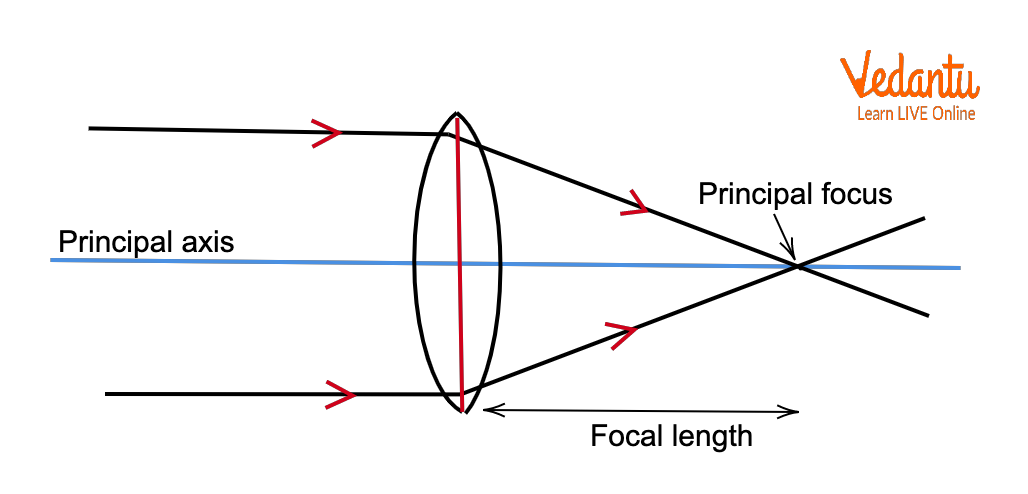Courses
Courses for Kids
Free study material
Free LIVE classes
More

# Sign Convention of Lens and Mirror for JEELIVE
Join Vedantu’s FREE Mastercalss

## What is Lens?

The lens in Physics is an optical device that is transmissive in nature and allows light rays to either focus at a point or disperse via refraction of light. The lens can be simple or compound. A simple lens has only one type of transparent material while a compound lens has multiple simple lenses combined or arranged over the same axis. There are two types of lenses - a convex lens and a concave lens as shown in the figure below.Schematic Diagram of a Concave and Convex Lens

## Properties of Lens

The following properties of the lens are important in order to solve any numerical,

• Index of Refraction: Refractive index of a lens is a dimensionless quantity which tells us about the ability of the lens to bend light, i.e., the capacity of refraction of light by the said optical device.

• Focal length: It is not an actual measurement of any length, but an approximate optical distance before the lens where the light rays combine to form a sharp image of an object kept a large distance away from the lens as shown in the figure below.The Schematic Diagram of the Convex Lens showing the Focal Length

• Principal Focus: The point on the principal axis at a distance equal to the focal length of the lens is called the principal focus.

• Radius of Curvature: The distance between the centre of the curved surface of the lens and the principal focus on the principal axis is known as the radius of curvature of the lens.

• Image Distance: The distance measured from the centre of a lens to the point where the image is formed is known as the image distance. When an object is placed at infinity, then the image formed by a convex lens is formed at the principal focus.

• Object Distance: The distance measured from the centre of the lens to the point where an object is placed is known as the object distance.

## New Cartesian Sign Convention of Spherical Lens

Now that we know the necessary parameters of the lens, we can determine their signs too in order to solve numerical problems. Sign convention is a collection of rules and is very important to understand as well as remember because without its knowledge, any numerical can give multiple wrong answers.

According to the rules,

1. All measurements of distances are done from the optical centre of the lenses.

2. The direction of the rays determines the signs of the parameter. The distances that are measured along the incident ray direction are positive. The distances that are measured in the opposite direction of the incident ray are negative.

3. The heights measured above the principal axis are positive. The heights measured below the principal axis are negative.

4. The focal length of the Convex lens is positive.

5. The focal length of the Concave lens is negative.

6. The object is always kept on the left side of the lens, so the distance of the object from the optical centre is negative.

7. The image distance is positive when the image forms right to the lens (real image) and negative when it forms on the left side of the lens (virtual image).

8. The object height is always along the positive y-axis, above the principal axis and so is positive.

9. The image can be erect or inverted and so the height is positive and negative, respectively.

## Sign Convention of Spherical Mirror

The points that define the sign convention of the mirror are listed below:

1. The focal length of the concave mirror is negative as the focus is on the left side.

2. The focal length of the convex mirror is negative as the focus is on the right side.

3. The distance of the object is always negative.

4. Image distance is negative if the image formed is real.

5. Image distance is positive if the image formed is virtual.

6. Object height is positive as it is always upright and above the principal axis.

7. Image height is negative if the image formed is inverted.

8. Image height is positive if the image formed is erect.

## Important Formulae for Numericals

Let us state and remember some of the most important lens formulae for solving the numerical: -

1. The lens formula is

$\frac{1}{v}-\frac{1}{u}=\frac{1}{f}$

Here, $v$ is the image distance, $u$ is the object distance, and $f$ is the focal length.

1. $f=\frac{v\times u}{u-v}$ is the focal length of a lens.

2. The Lens maker’s formula is

$\frac{1}{f}=\left( n-1 \right)\left( \frac{1}{{{R}_{1}}}+\frac{1}{{{R}_{2}}} \right)$

Here, $n$ is the refractive index, and the $Rs$ is the radii of curvature of the lens.

1. Magnification is expressed as:

$m=\frac{\text{image height}}{\text{object height}}$

$m=\frac{v}{u}$

## Conclusions

Lens is an optical device capable of light ray focusing or dispersing via refraction. Convex lenses converge light rays while concave lenses diverge light rays. Convex lenses form both real and virtual images whereas concave lenses form only virtual images. The object distance is negative in both lenses. In convex lenses, focus and the real image are on the right side of the lens and the real image is inverted in nature. However, there is no virtual focus in convex lenses and an upright virtual image is formed on the left side of the lens.

In a concave lens, the virtual image and virtual focus both are on the left hand side of the lens and the image formed is upright in nature.

Last updated date: 21st Sep 2023
Total views: 123.6k
Views today: 2.23k

## FAQs on Sign Convention of Lens and Mirror for JEE

1. An object is placed at infinity. Where is the image formed by a convex lens? What will happen if the lens is concave?

The image will be formed at the principal focus. For convex lenses, if any object is placed at infinite distances, then the image will be formed at a distance equal to the focal length of the convex lens.  For a concave lens, the image formed will be virtual and it will be formed right at the focus of the lens. The image size will be highly diminished as compared to the object size and can be called a point image.

2. Why and how does the lens bend light?

We know that every material is made of electrons. Now, when two matter interact, the electrons will also undergo the interaction and their behaviour is manifested as the phenomenon we get to observe. So, when the light passes closely around any material, the electrons inside the material get strongly affected and the influence gives the light rays a bent trajectory. The measure of how much a material can bend a light ray is expressed via its refractive index.